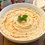# Proof of Minkowski's inequality

this follows from Holder's inequality,and in my proof,for the sake of simplicity,i'll use it

Minkowski's inequality states that

$$\displaystyle\sum _{ n=1 }^{ k }{ ({ x }_{ n }+{ y }_{ n })^{ \frac { 1 }{ p } }\le (\displaystyle\sum _{ n=1 }^{ k }{ { x }_{ n }^{ p } } ) } ^{ \frac { 1 }{ p } }+(\displaystyle\sum _{ n=1 }^{ k }{ { y }_{ n }^{ p } } )^{ \frac { 1 }{ p } }$$

for $p>1$

and ${x}_{n},{y}_{n}\ge0$

Proof:

we know

$\displaystyle\sum _{ n=1 }^{ k }{ ({ x }_{ n }+{ y }_{ n })^{ p }=\displaystyle\sum _{ n=1 }^{ k }{ { x }_{ n }({ x }_{ n }+{ y }_{ n })^{ p-1 } } } +\displaystyle\sum _{ n=1 }^{ k }{ { y }_{ n }({ x }_{ n }+{ y }_{ n } } )^{ p-1 }$

let's define $a$ as $a=\frac { p }{ p-1 }$ now by Holder's inequality we have

$\displaystyle \sum_{n \mathop = 1}^k x_n \left({x_n + y_n}\right)^{p-1} + \sum_{n \mathop = 1}^k y_n \left({x_n + y_n}\right)^{p-1}\le((\sum _{ n=1 }^{ k }{ { x }_{ n }^{ p } } )^{ \frac { 1 }{ p } }+(\sum _{ n=1 }^{ k }{ { y }_{ n }^{ p } } )^{ \frac { 1 }{ p } })((\sum _{ n=1 }^{ k }{ ({ x }_{ k }+{ y }_{ k })^{ p })^{ \frac { 1 }{ a } } } )=\$$(\displaystyle\sum _{ n=1 }^{ k }{ { x }_{ n }^{ p } } )^{ \frac { 1 }{ p } }+(\displaystyle\sum _{ n=1 }^{ k }{ { y }_{ n }^{ p } } )^{ \frac { 1 }{ p } })(\displaystyle\sum _{ n=1 }^{ k }{ ({ x }_{ n }+{ y }_{ n })^{ p } } )^{ \frac { 1 }{ a } }$ dividing by $\displaystyle\sum _{ n=1 }^{ k }{ ({ x }_{ n }+{ y }_{ n })^{ p } } )^{ \frac { 1 }{ a } }$ we get $\displaystyle\sum _{ n=1 }^{ k }{ ({ x }_{ n }+{ y }_{ n })^{ \frac { 1 }{ p } }\le (\displaystyle\sum _{ n=1 }^{ k }{ { x }_{ n }^{ p } } ) } ^{ \frac { 1 }{ p } }+(\displaystyle\sum _{ n=1 }^{ k }{ { y }_{ n }^{ p } } )^{ \frac { 1 }{ p } }$ Hence provedNote by Hamza A 5 years, 5 months ago This discussion board is a place to discuss our Daily Challenges and the math and science related to those challenges. Explanations are more than just a solution — they should explain the steps and thinking strategies that you used to obtain the solution. Comments should further the discussion of math and science. When posting on Brilliant: • Use the emojis to react to an explanation, whether you're congratulating a job well done , or just really confused . • Ask specific questions about the challenge or the steps in somebody's explanation. Well-posed questions can add a lot to the discussion, but posting "I don't understand!" doesn't help anyone. • Try to contribute something new to the discussion, whether it is an extension, generalization or other idea related to the challenge. • Stay on topic — we're all here to learn more about math and science, not to hear about your favorite get-rich-quick scheme or current world events. MarkdownAppears as *italics* or _italics_ italics **bold** or __bold__ bold - bulleted- list • bulleted • list 1. numbered2. list 1. numbered 2. list Note: you must add a full line of space before and after lists for them to show up correctly paragraph 1paragraph 2 paragraph 1 paragraph 2 [example link](https://brilliant.org)example link > This is a quote This is a quote  # I indented these lines # 4 spaces, and now they show # up as a code block. print "hello world" # I indented these lines # 4 spaces, and now they show # up as a code block. print "hello world" MathAppears as Remember to wrap math in \( ... $$ or $ ... $ to ensure proper formatting.
2 \times 3 $2 \times 3$
2^{34} $2^{34}$
a_{i-1} $a_{i-1}$
\frac{2}{3} $\frac{2}{3}$
\sqrt{2} $\sqrt{2}$
\sum_{i=1}^3 $\sum_{i=1}^3$
\sin \theta $\sin \theta$
\boxed{123} $\boxed{123}$

Sort by:

Can someone clearly prove for what conditions do we have the equality?

- 4 years, 10 months ago

very good job dude :O

- 5 years, 5 months ago

thanks :)

i tried 2 proofs,one was just too long,so i went with the simpler one :)

- 5 years, 5 months ago

Great proof!!!!

- 5 years, 5 months ago

thanks!

- 5 years, 5 months ago

You should post your findings in the wiki page, hölder's inequality.

- 5 years, 5 months ago

Give introduction to minkowski's inequality

- 2 years, 10 months ago

The term to the left in your result should be [sum((xn+yn)^p)]^(1/p), so what you have written is wrong here as a result.

- 10 months, 4 weeks ago